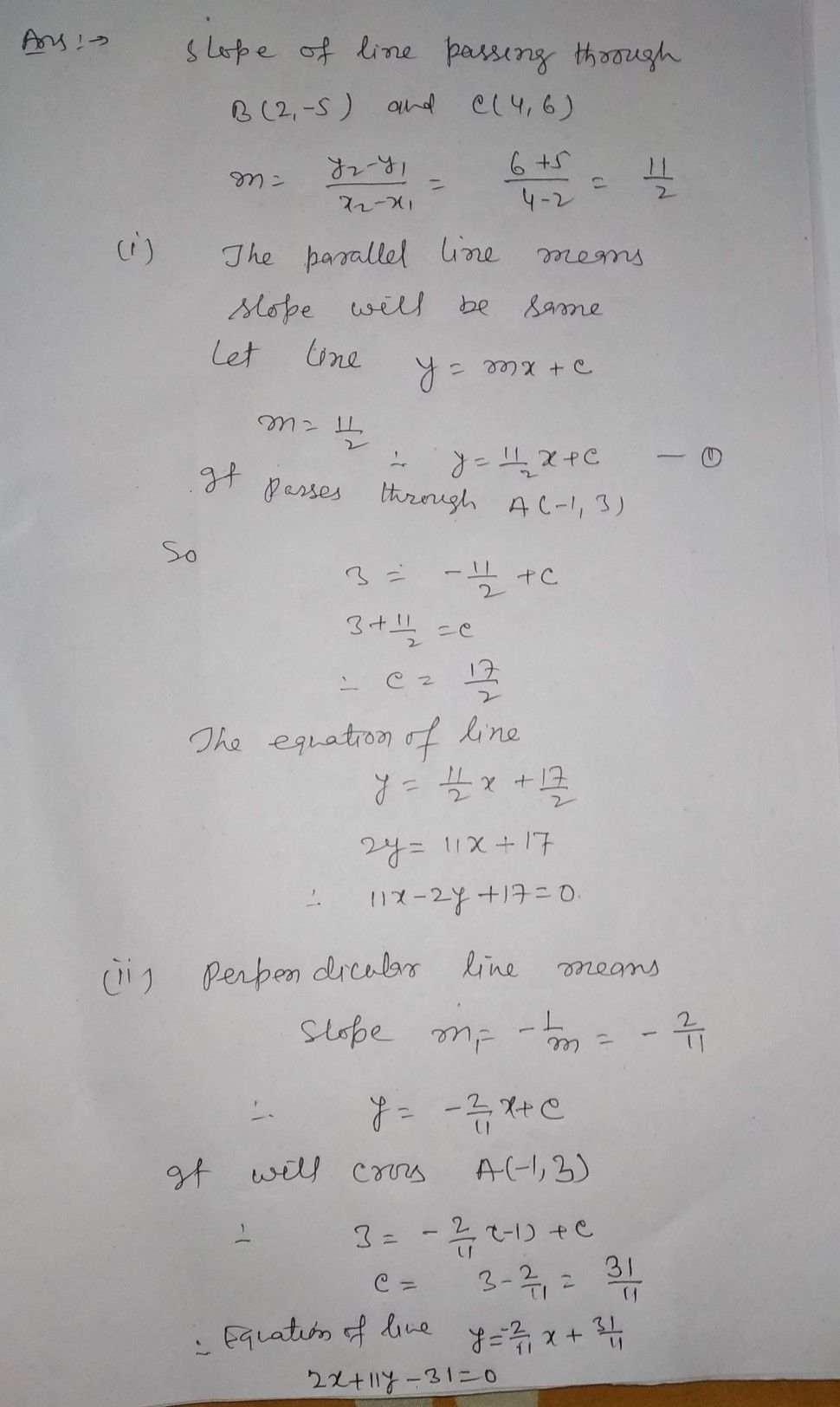Symbol
ProblemThe Straight Line $3,$ $P\pi 0blem$ Find the equation of the straight line passing through $A\left(-1,3\right)$ and $\left(1\right)$ para $\left(11\right)$ perpendicular to the straight line passing through $B\left(2,$ $-5\right)$ $S$ $-$ $C$ $\left(4,6\right)$ $6+5$ $11$
Calculus
Question content
find
SolutionQanda teacher - MOHD HOMAI